# Relaxation method

(diff) ← Older revision | Latest revision (diff) | Newer revision → (diff)

weakening method

A method for the iterative solution of a system of linear algebraic equations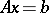, the elementary step of which consists of varying only one component of the vector of unknowns, the number of variable components being chosen in a specific cyclic order. The relaxation method is most often used for solving systems with a positive-definite matrix.

If one component of the vector of unknowns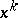is varied such that for the new approximation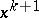the quadratic formis minimized, then the relaxation method is called a complete relaxation method. If, however, after one elementary step the value of the quadratic form is only reduced and not minimized, the relaxation method is called an incomplete relaxation method.

The best investigated method is that of successive upper relaxation, where the matrixpossesses the so-called property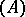and is ordered accordingly. A matrixis called a matrix possessing propertyif there is a permutation matrixsuch that the matrix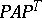has the form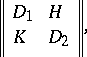where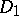and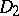are square diagonal matrices.

The iteration scheme of the relaxation method is as follows: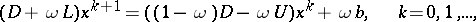whereis the relaxation parameter,is the diagonal,is the lower-triangular andis the upper-triangular matrix in the decomposition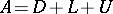. If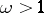, then the method is called an upper relaxation method (over-relaxation), and if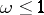, a lower relaxation method. The parameteris chosen from the condition of minimization of the spectral radius of the matrixof transfer from iteration to iteration: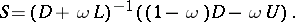Ifis a symmetric matrix with positive diagonal elements and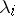are the roots of the determinant equation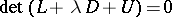, then the optimum value of the parameteris given by the formulawhere. Forthe spectral radius ofis equal toCases are examined where someare complex. Block relation methods have been developed.

How to Cite This Entry:
Relaxation method. Encyclopedia of Mathematics. URL: http://encyclopediaofmath.org/index.php?title=Relaxation_method&oldid=17189
This article was adapted from an original article by E.S. Nikolaev (originator), which appeared in Encyclopedia of Mathematics - ISBN 1402006098. See original article